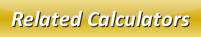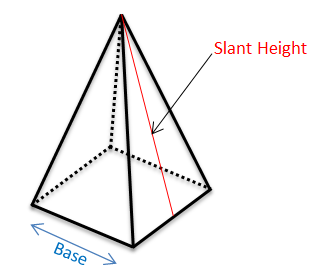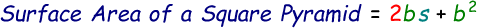# Surface Area of a Square Pyramid Calculator

A normal pyramid would possess 3 sides and a tip called apex, whereas the square pyramid is pretty similar except it contains 4 sides and an apex.ĀWe can calculate the surface area of a square pyramid when we know the slant height and base length. Once we know these parameters we can calculate surface area with the help of this below formula:where,
b = Base Length
s = Slant Height

Enter the slant height and base length in the below online surface area of a square pyramid calculator and press calculate to find the result.

 Base Length of Square Pyramid (b): Slant Height of Square Pyramid (s): Surface Area of Square Pyramid:

Latest Calculator Release

Average Acceleration Calculator

Average acceleration is the object's change in speed for a specific given time period. ...

Free Fall Calculator

When an object falls into the ground due to planet's own gravitational force is known a...

Torque Calculator

Torque is nothing but a rotational force. In other words, the amount of force applied t...

Average Force Calculator

Average force can be explained as the amount of force exerted by the body moving at giv...

Angular Displacement Calculator

Angular displacement is the angle at which an object moves on a circular path. It is de...